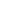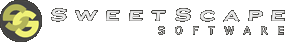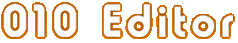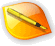Edit AnythingProfessional text and hex editingwith Binary Templates technology.010 Editor - Text/Hex Editor Homepage

 Math FunctionsThe following is a list of math functions that can be used when writing Templates or Scripts.double Abs( double x )
Returns the absolute value of the float pointer number x.double Ceil( double x )
Returns the smallest integer not less than x.double Cos( double a )
Returns the cosine of the given angle. The angle is given in degrees.double Exp( double x )
Calculates the exponential e to the power of x.double Floor( double x)
Returns the highest integer less than or equal to x.double Log( double x )
Calculates the natural logarithm of x. This value is also known as ln(x).double Log10( double x )
Calculates the common logarithm of x, also called the base-10 logarithm. For example, Log10(1000)=3.

Requires 010 Editor v12.0 or higher.double Max( double a, double b )
Returns the larger of the numbers a and b.double Min( double a, double b)
Returns the smaller of the numbers a and b.double Pow( double x, double y)
Returns x to the power of y.int Random( int maximum )
Returns a random integer between 0 and maximum-1 inclusive.double Sin( double a )
Computes the sine of the given angle. The angle is given in degrees.double Sqrt( double x )
Calculates the positive square-root of the number x.data_type SwapBytes( data_type x )
Swaps the bytes of the variable and returns the result. Any of the basic data types can be specified (byte, short, int, int64, float, or double).double Tan( double a )
Calculates the tangent of the given angle. The angle is given in degrees.Copyright © 2003-2013 SweetScape Software - www.sweetscape.com

This is the manual for 010 Editor, a professional hex editor and text editor. Use 010 Editor to edit the individual bytes of any binary file, hard drive, or process on your machine. 010 Editor contains a whole host of powerful analysis and editing tools, plus Binary Templates technology that allows any binary format to be understood.Newsletter - Receive special offers, tips, tricks and news. Join now010 Editor v14.0 is here! What's new?NavigationProducts010 Editor